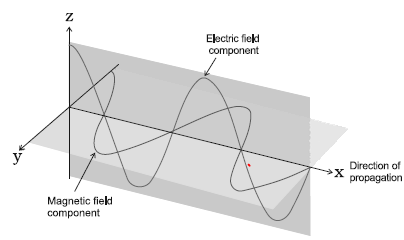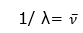## Electromagnetic Theory:-

This theory was given by Clark Maxwell
Main points are:-
i) When an electrically charged particle moves under acceleration, alternating electrical and magnetic fields are produced and transmitted. These fields are transmitted in the form of waves. These waves are called electromagnetic waves or electromagnetic radiations
ii) Energy emitted from any source continuously the form of radiations & is called radiant energy.
iii)  The radiation consists of electric & magnetic fields oscillating perpendicular to each other & both perpendicular to direction of propagation of radiation.
iv) The radiation possesses wave character & travel with the speed of light (3 X 108 m/s)
v) These waves do not require any meteoric medium for propagation.## Characteristic of wave:-

### WAVELENGTH

The distance b/w two consecutive crest or troughs (λ)

### Frequency

The no. of waves or cycles travelling per second is frequency.
ν = 1/T

### Amplitude

It is the height of crust or trough. It is represented by ‘a’.

### Velocity

Displacement per unit time m/s.  In vacuum all types of electromagnetic radiations travel with the same velocity. Its value is 3 X108 m/s.

### Wave no: (v)

It is reciprocal of wavelength### Important relationship between Speed of light, wavelength and Frequency is given by

C = νλ
Here C = 3 X108 m/s. = Light of speed
Question 1
Yellow light emitted from sodium lamp have wave length 580 nm. Calculate Frequency and Wave Number
Solution
Given: Wavelength = 580 nm
= 580 X 10-9   m
C = 3 X108 m/s
As,   C = ν λ
ν = λ/C = 580 X 10-9   / 3 X108
=5.5 X 1014 /sec

Question 2
calculate the frequency of light having wavelength 0.05 A0
Solution
Given:
Wavelength = 0.05 A0= .05 × 10-10 m
Now, C = νλ
ν =C/λ
=3 × 108/ .05 × 10-10
=6 × 1019 /sec

Question 3
Calculate wavelength,frequency and wave no. of wave whose time period is 2 × 10-10 s
Solution
time period =2 × 10-10 s

(i) Frequency= 1/ T = 1/2 × 10-10 = .5 × 1010 sec-1
(ii) C = νλ
λ= c /ν = 3 × 108/.5 × 109=6 X 10-1 m
(iii) Now wave no. = 1/λ
= 16.667 m-1 (approx.)

## Electromagnetic Spectrum-

When magnetic radiation are arranged in order of increasing wavelength or decreasing frequencies, the complete spectrum obtained is known as electromagnetic spectrum.
Visible region is from 400 nm to 150 nm.

## Limitations of Electromagnetic wave theory:-

1. This theory doesn’t explain the phenomenon of photoelectric effect.
2. It doesn’t explain of black body radiation.
3. It doesn’t explain line spectrum of atom with respect to hydrogen.